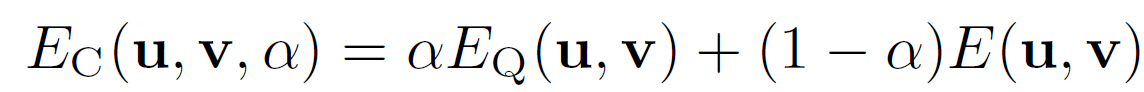Optimization of energy terms can be difficult in OF, because of non-convexity and local optima. Construct a series of energy functionsEQ is convex, quadratic alpha changes from 1 to 0, so Energy Ec changes from quadratic to original. for each alpha they find optimum through setting derivatives of Ec to 0. Solution on each stage becomes initialization on the next one.

Proposed in: D. Sun, S. Roth, J. Lewis, and M. J. Black. Learning optical flow. In ECCV, volume 3, pages 83–97, 2008. [[pdf](http://cs.brown.edu/~dqsun/pubs/eccv2008.pdf" target="_blank)]